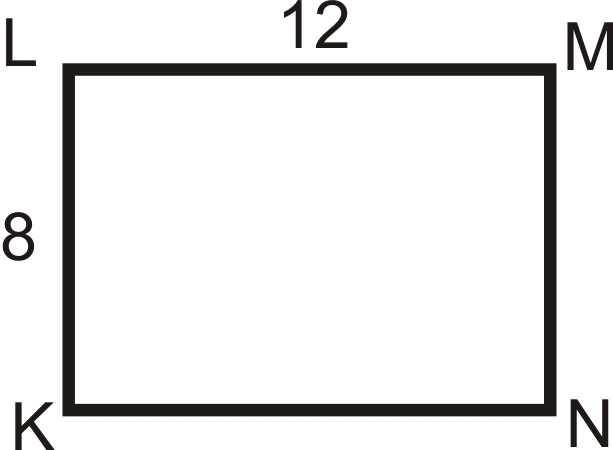# 7.15: Dilation of a Shape

•• Contributed by CK12
• CK12

Larger or smaller version of a figure that preserves its shape.

## Dilation

Two figures are similar if they are the same shape but not necessarily the same size. One way to create similar figures is by dilating. A dilation makes a figure larger or smaller but the new resulting figure has the same shape as the original.

Dilation: An enlargement or reduction of a figure that preserves shape but not size. All dilations are similar to the original figure.

Dilations have a center and a scale factor. The center is the point of reference for the dilation and the scale factor tells us how much the figure stretches or shrinks. A scale factor is labeled $$k$$. Only positive scale factors, $$k$$, will be considered in this text.

If the dilated image is smaller than the original, then $$0<k<1$$.

If the dilated image is larger than the original, then $$k>1$$.

A dilation, or image, is always followed by a $$′$$.

Label It Say It
$$′$$ “prime” (copy of the original)
$$A′$$ “a prime” (copy of point $$A$$)
$$A′′$$ “a double prime” (second copy)

What if you enlarged or reduced a triangle without changing its shape? How could you find the scale factor by which the triangle was stretched or shrunk?

Example $$\PageIndex{1}$$

Find the perimeters of $$KLMN$$ and $$K′L′M′N′$$. Compare this ratio to the scale factor.Figure $$\PageIndex{1}$$

Solution

The perimeter of $$KLMN=12+8+12+8=40$$. The perimeter of $$K′L′M′N′=24+16+24+16=80$$. The ratio is 80:40, which reduces to 2:1, which is the same as the scale factor.

Example $$\PageIndex{2}$$Figure $$\PageIndex{2}$$

$$\Delta ABC$$ is a dilation of $$\Delta DEF$$. If P is the center of dilation, what is the scale factor?

SolutionFigure $$\PageIndex{3}$$

Because $$\Delta ABC$$ is a dilation of $$\Delta DEF$$, then $$\Delta ABC\sim \Delta DEF$$. The scale factor is the ratio of the sides. Since $$\Delta ABC$$ is smaller than the original, $$\Delta DEF$$, the scale factor is going to be less than one, $$\dfrac{12}{20}=\dfrac{3}{5}$$.

If $$\Delta DEF$$ was the dilated image, the scale factor would have been $$\dfrac{5}{3}$$.

Example $$\PageIndex{3}$$

The center of dilation is $$P$$ and the scale factor is 3.

Find $$Q′$$.Figure $$\PageIndex{4}$$

Solution

If the scale factor is 3 and $$Q$$ is 6 units away from $$P$$, then $$Q′$$ is going to be $$6\times 3=18$$ units away from $$P$$. The dilated image will be on the same line as the original image and center.Figure $$\PageIndex{5}$$

Example $$\PageIndex{4}$$

Using the picture above, change the scale factor to 13.

Find $$Q′′$$ using this new scale factor.

Solution

The scale factor is $$\dfrac{1}{3}$$, so $$Q′′$$ is going to be $$6\times \dfrac{1}{3}=2$$ units away from $$P$$. $$Q′′$$ will also be collinear with $$Q$$ and center.Figure $$\PageIndex{6}$$

Example $$\PageIndex{5}$$

$$KLMN$$ is a rectangle. If the center of dilation is $$K$$ and $$k=2$$, draw $$K′L′M′N′$$.Figure $$\PageIndex{7}$$

Solution

If $$K$$ is the center of dilation, then $$K$$ and $$K′$$ will be the same point. From there, $$L′$$ will be 8 units above $$L$$ and $$N′$$ will be 12 units to the right of $$N$$.Figure $$\PageIndex{8}$$

## Review

For the given shapes, draw the dilation, given the scale factor and center.

1. $$k=3.5$$, center is $$A$$Figure $$\PageIndex{9}$$
1. $$k=2$$, center is $$D$$Figure $$\PageIndex{10}$$
1. $$k=\dfrac{3}{4}$$, center is $$A$$Figure $$\PageIndex{11}$$
1. $$k=\dfrac{2}{5}$$, center is A\)Figure $$\PageIndex{12}$$

In the four questions below, you are told the scale factor. Determine the dimensions of the dilation. In each diagram, the black figure is the original and $$P$$ is the center of dilation.

1. $$k=4$$Figure $$\PageIndex{13}$$
1. $$k=\dfrac{1}{3}$$Figure $$\PageIndex{14}$$
1. $$k=2.5$$Figure $$\PageIndex{15}$$
1. $$k=\dfrac{1}{4}$$Figure $$\PageIndex{16}$$

In the three questions below, find the scale factor, given the corresponding sides. In each diagram, the black figure is the original and $$P$$ is the center of dilation.

1.Figure $$\PageIndex{17}$$
2.Figure $$\PageIndex{18}$$
3.Figure $$\PageIndex{19}$$

## Vocabulary

Term Definition
Dilation To reduce or enlarge a figure according to a scale factor is a dilation.
Quadrilateral A quadrilateral is a closed figure with four sides and four vertices.
Ratio A ratio is a comparison of two quantities that can be written in fraction form, with a colon or with the word “to”.
Scale Factor A scale factor is a ratio of the scale to the original or actual dimension written in simplest form.
Transformation A transformation moves a figure in some way on the coordinate plane.
Vertex A vertex is a point of intersection of the lines or rays that form an angle.
Rigid Transformation A rigid transformation is a transformation that preserves distance and angles, it does not change the size or shape of the figure.

Interactive Element

Video: Dilation Principles - Basic

Activities: Dilation Discussion Questions

Study Aids: Types of Transformations Study Guide

Practice: Dilation of a Shape

Real World: The CSI Effect